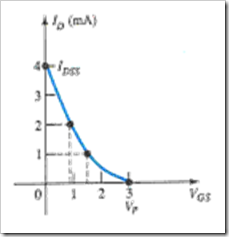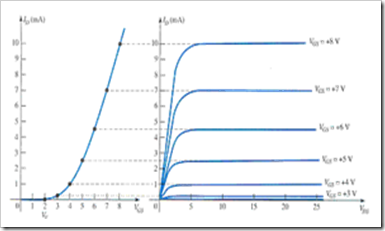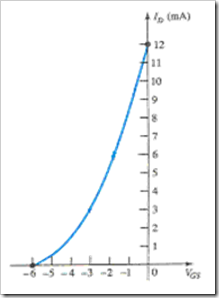# Practice Quiz in Field Effect Transistor Devices Part 2

(Last Updated On: February 3, 2020)

This is the Online Practice Quiz in Field Effect Transistor Devices Part 2 from the book, Electronic Devices and Circuit Theory 10th Edition by Robert L. Boylestad. If you are looking for a reviewer in Electronics Engineering this will definitely help. I can assure you that this will be a great help in reviewing the book in preparation for your Board Exam. Make sure to familiarize each and every questions to increase the chance of passing the ECE Board Exam.

### Continue Part II of the Online Practice Quiz

#### Quiz in Field Effect Transistor Devices

Question 11. The BJT is a _____ device. The FET is a _____ device.

A. bipolar, bipolar

B. bipolar, unipolar

C. unipolar, bipolar

D. unipolar, unipolar

Question 12. What is the ratio of ID / IDSS for VGS = 0.5 VP?

A. 0.25

B. 0.5

C. 1

D. 0

Question 13. Referring to this transfer curve, determine ID at VGS = 2 V.A. 0.444 mA

B. 1.333 mA

C. 0.111 mA

D. 4.444 mA

Question 14. Which of the following applies to a safe MOSFET handling?

A. Always pick up the transistor by the casing.

B. Power should always be off when network changes are made.

C. Always touch ground before handling the device.

D. All of these

Question 15. Refer to the following figure. Calculate VGS at ID = 8 mA for k = 0.278 × 10–2 A/V2.A. 3.70 V

B. 5.36 V

C. 7.36 V

D. 2.36 V

Question 16. Hand-held instruments are available to measure _____ for the BJT.

A. βdc

B. IDSS

C. VP

D. All of these

Question 17. What is the level of IG in an FET?

A. Zero amperes

B. Equal to ID

C. Depends on VDS

D. Undefined

Question 18. The level of VGS that results in ID = 0 mA is defined by VGS = _____.

A. VGS(off)

B. VP

C. VDS

D. None of these

Question 19. Which of the following ratings appear(s) in the specification sheet for an FET?

A. Voltages between specific terminals

B. Current levels

C. Power dissipation

D. All of these

Question 20. Referring to this transfer curve. Calculate (using Shockley's equation) VGS at ID = 4mA.A. 2.54 V

B. –2.54 V

C. –12 V

D. Undefined

### More Practice Quiz in Field Effect Transistor Devices

Practice Quiz Part 1

Practice Quiz Part 2

Practice Quiz Part 3

Practice Quiz Part 4

Practice Quiz Part 5

Practice Quiz Part 6

### See: Complete List of Practice Quizzes

Note: After taking this particular quiz, you can proceed to check all the topics.